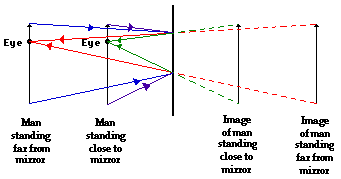# Question #feb5aSo the minimum length of the mirror required to see the full image of the girl of height 160cm should be half of her image's height i.e.$= \frac{1}{2} \times 160 c m = 80 c m$
The lower end of the mirror will be at the half the height of girl's eye from the floor and it will be $G M = \frac{1}{2} \times E L = \frac{1}{2} \times 152 c m = 76 c m$